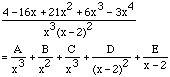Date: Sat, 11 Jul 1998 06:35:15 -0700
Subject: Calculus problems

I'm stuck again. Can you help?

This involves integration using the method of partial fractions

the integral of:
7x(to the 5th) - 2x(cubed) + 3 dx
--------------------------------------
x(to the fourth) - 81

Do I have to do long division to reduce the numerator to the fourth power?

the integral of:
4- 16x +21x(squared) + 6x(cubed) - 3x(fourth) dx
----------------------------------------------------
x(cubed)(x - 2)(squared)

Lorraine
correspondence student